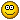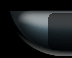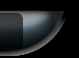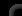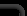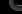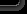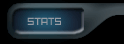76191 Posts in 13394 Topics by 2073 Members - Latest Member: caparuni February 19, 2020, 07:15:35 am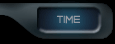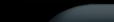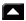|Home|Help|Search|Login|Register|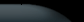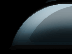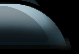###Author Topic: more admin auto login with display pic  (Read 1852 times)

0 Members and 1 Guest are viewing this topic.

####ÞØÚÑÐËR

• Forum Member« on: October 23, 2006, 04:11:00 pm »
what i have done is added more pics and now have welcome pic for regular users..as it is..it works..but when an admin enters..it displays both reguar user and there own pic. how would you make it so only the right pic displays for the admin...anyone??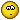Code
``<OnEnter type="random"> <out type="self" condition="1" lvalue="%IP%" rvalue="%IP%">!pic1</out> <out type="self" condition="1" lvalue="%IP%" rvalue="%IP%">!pic2</out> <out type="self" condition="1" lvalue="%IP%" rvalue="%IP%">!pic3</out> </OnEnter> <OnEnter type="script"> <out delay="0000" condition="1" lvalue="%IP%" rvalue="(IP1 HERE)">/forcelogin %NAME% (LOGIN HERE)</out> <out delay="0000" condition="1" lvalue="%IP%" rvalue="(IP2 HERE)">/forcelogin %NAME% (LOGIN HERE)</out> <out delay="0000" condition="1" lvalue="%IP%" rvalue="(IP3 here)">/forcelogin %NAME% (LOGIN HERE)</out> <out type="self" condition="1" lvalue="%IP%" rvalue="(IP1 HERE)">!picture1</out> <out type="self" condition="1" lvalue="%IP%" rvalue="(IP2 HERE)">!picture2</out> <out type="self" condition="1" lvalue="%IP%" rvalue="(IP3 here)">!picture3</out> </OnEnter> <command type="script" codition="1" lvalue="%PARAM%" rvalue="_BOT_SELF_TRIGGER_"> <in>!pic1</in> <out>(PIC HERE)</out> </command> <command type="script" codition="1" lvalue="%PARAM%" rvalue="_BOT_SELF_TRIGGER_"> <in>!pic2</in> <out>(PIC HERE)</out> </command> <command type="script" codition="1" lvalue="%PARAM%" rvalue="_BOT_SELF_TRIGGER_"> <in>!pic3</in> <out>(PIC HERE)</out> </command> <command type="script" codition="1" lvalue="%PARAM%" rvalue="_BOT_SELF_TRIGGER_"> <in>!picture1</in> <out>(PIC HERE)</out> </command> <command type="script" codition="1" lvalue="%PARAM%" rvalue="_BOT_SELF_TRIGGER_"> <in>!picture2</in> <out>(PIC HERE)</out> </command> <command type="script" codition="1" lvalue="%PARAM%" rvalue="_BOT_SELF_TRIGGER_"> <in>!picture3</in> <out>(PIC HERE)</out> </command>``

As you see i have change the script a little from the earlyer posting...i remover the member group and just went by ip (as sugested) and seems to give much better results..so ty for that helpful hint also...chears

####Max™

• MX Hosts
•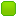•• If Im Not Back later... Wait Longer« Reply #1 on: October 24, 2006, 09:31:33 am »
well looking at it, hers what i think could work, but i havent tested it, i'll give you that pleasure
Code
``where you have this<command type="script" codition="1" lvalue="%PARAM%" rvalue="_BOT_SELF_TRIGGER_"><in>!pic1</in><out>(PIC HERE)</out></command> the out could be limited to an ip so that pic will only work on that given ip like this<command type="script" codition="1" lvalue="%PARAM%" rvalue="_BOT_SELF_TRIGGER_"><in>!pic1</in><out condition="1" lvalue="%IP%" rvalue="(IP2 HERE)">(PIC HERE)</out></command> ``

Try Connecting, the attacks may let you https://patch.winmxconex.com/

####ÞØÚÑÐËR

• Forum Member« Reply #2 on: October 25, 2006, 06:57:38 pm »
thx for the reply...it does give me a few ideas to try... but ...
This part of the scropt for the admins works great..directs the right ip to the right pic

Code
``<OnEnter type="script"> <out delay="0000" condition="1" lvalue="%IP%" rvalue="(IP1 HERE)">/forcelogin %NAME% (LOGIN HERE)</out> <out delay="0000" condition="1" lvalue="%IP%" rvalue="(IP2 HERE)">/forcelogin %NAME% (LOGIN HERE)</out> <out delay="0000" condition="1" lvalue="%IP%" rvalue="(IP3 here)">/forcelogin %NAME% (LOGIN HERE)</out> <out type="self" condition="1" lvalue="%IP%" rvalue="(IP1 HERE)">!picture1</out> <out type="self" condition="1" lvalue="%IP%" rvalue="(IP2 HERE)">!picture2</out> <out type="self" condition="1" lvalue="%IP%" rvalue="(IP3 here)">!picture3</out> </OnEnter> ``

the problem is with the random part...

Code
``<OnEnter type="random"> <out type="self" condition="1" lvalue="%IP%" rvalue="%IP%">!pic1</out> <out type="self" condition="1" lvalue="%IP%" rvalue="%IP%">!pic2</out> <out type="self" condition="1" lvalue="%IP%" rvalue="%IP%">!pic3</out> </OnEnter>``

being it is random it also picks up our ip and displays a regular user pic as well..is there so way to make this part ignore our ip's..or to maybe stop the script if our ip's are found??

Im still new to this so pardon my ignorance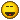####Max™

• MX Hosts
••• If Im Not Back later... Wait Longer« Reply #3 on: October 25, 2006, 07:45:16 pm »
yes there is a way it can ignore the admin ip's
u add a usergroup to it as shown below but pit an excamation mark  !  infromt of the admin group, this tells the bot to ignore any admin in the admins usergroup, a normal admin only group would be usergroup="admins"  to exclude an admin group is usergroup="!admins"

Code
``<OnEnter type="random" usergrpou="!admins"><out type="self" condition="1" lvalue="%IP%" rvalue="%IP%">!pic1</out><out type="self" condition="1" lvalue="%IP%" rvalue="%IP%">!pic2</out><out type="self" condition="1" lvalue="%IP%" rvalue="%IP%">!pic3</out></OnEnter>``

Try Connecting, the attacks may let you https://patch.winmxconex.com/

####ÞØÚÑÐËR

• Forum Member« Reply #4 on: October 26, 2006, 12:03:47 am »
well i got the jist of what your saying and have tryed a few dozen things but still no luck.  how about another friendly pointer...am i warm...lmao

Code
``<usergroup name="admins"> !USER1</usergroup> <OnEnter type="random" usergrpou="!admins"><out type="self" condition="1" lvalue="%NAME%" rvalue="%NAME%">pic1</out><out type="self" condition="1" lvalue="%NAME%" rvalue="%NAME%">pic2</out><out type="self" condition="1" lvalue="%NAME%" rvalue="%NAME%">pic3</out></OnEnter><OnEnter type="script"><out delay="1000" condition="1" lvalue="%IP%" rvalue="(ip1)">/forcelogin %NAME (LOGIN)</out><out delay="1000" condition="1" lvalue="%IP%" rvalue="(ip2)">/forcelogin %NAME% (LOGIN_</out><out delay="1000" condition="1" lvalue="%IP%" rvalue="(IP3)">/forcelogin %NAME% (LOGIN)</out><out type="self" condition="1" lvalue="%IP%" rvalue="(IP1)">!pic4</out><out type="self" condition="1" lvalue="%IP%" rvalue="(ip2)">!pic5</out> <out type="self" condition="1" lvalue="%IP%" rvalue="(IP3)">!pic6</out></OnEnter>``

####ÞØÚÑÐËR

• Forum Memberok..after lots of trial and error..i finally got it to work..ty for you help..chears